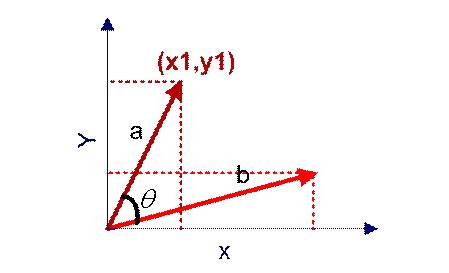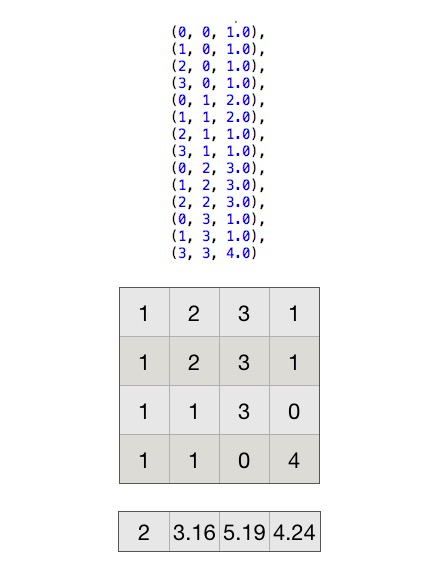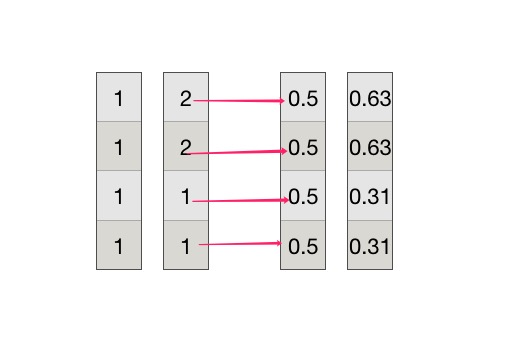# 相似度

• 欧氏距离，在几何中最简单的计算方法
• 夹角余弦，通过方向计算相似度，通常在用户对商品评分、NLP等场景使用
• 杰卡德距离，在不考虑每一样的具体值时使用
• 皮尔森系数，与夹角余弦类似，但是可以去中心化。比如评分时，有人倾向于打高分，有人倾向于打低分，他们的最后效果在皮尔森中是一样的
• 曼哈顿距离，一般在路径规划、地图类中常用，比如A*算法中使用曼哈顿来作为每一步代价值的一部分（F=G+H, G是从当前点移动到下一个点的距离，H是距离目标点的距离，这个H就可以用曼哈顿距离表示）$\left( { x }_{ 1 },{ y }_{ 1 } \right)$

$\left( { x }_{ 2 },{ y }_{ 2 } \right)$

$cos(\theta )=\frac { a\cdot b }{ ||a||\ast ||b|| } \\ =\quad \frac { { x }_{ 1 }\ast { x }_{ 2 }\quad +\quad { y }_{ 1 }\ast y_{ 2 } }{ \sqrt { { x }_{ 1 }^{ 2 }+{ x }_{ 2 }^{ 2 } } \ast \sqrt { { y }_{ 1 }^{ 2 }+{ y }_{ 2 }^{ 2 } } }$

# 公式拆解

$第一个向量：({x}_{1}, {x}_{2}, {x}_{3}, ..., {x}_{n})\\ 第二个向量：({y}_{1}, {y}_{2}, {y}_{3}, ..., {y}_{n})$

$cos(\theta )\quad =\quad \frac { \sum _{ i=1 }^{ n }{ ({ x }_{ i }\ast { y }_{ i }) } }{ \sqrt { \sum _{ i=1 }^{ n }{ { x }_{ i }^{ 2 } } } \ast \sqrt { \sum _{ i=1 }^{ n }{ y_{ i }^{ 2 } } } } \\ =\quad \frac { { x }_{ 1 }\ast { y }_{ 1 }+{ x }_{ 2 }\ast { y }_{ 2 }+...+{ x }_{ n }\ast { y }_{ n } }{ \sqrt { \sum _{ i=1 }^{ n }{ { x }_{ i }^{ 2 } } } \ast \sqrt { \sum _{ i=1 }^{ n }{ y_{ i }^{ 2 } } } } \\ =\quad \frac { { x }_{ 1 }\ast { y }_{ 1 } }{ \sqrt { \sum _{ i=1 }^{ n }{ { x }_{ i }^{ 2 } } } \ast \sqrt { \sum _{ i=1 }^{ n }{ y_{ i }^{ 2 } } } } +\frac { { x }_{ 2 }\ast { y }_{ 2 } }{ \sqrt { \sum _{ i=1 }^{ n }{ { x }_{ i }^{ 2 } } } \ast \sqrt { \sum _{ i=1 }^{ n }{ y_{ i }^{ 2 } } } } +...+\frac { { x }_{ n }\ast { y }_{ n } }{ \sqrt { \sum _{ i=1 }^{ n }{ { x }_{ i }^{ 2 } } } \ast \sqrt { \sum _{ i=1 }^{ n }{ y_{ i }^{ 2 } } } } \\ =\quad \frac { { x }_{ 1 } }{ \sqrt { \sum _{ i=1 }^{ n }{ { x }_{ i }^{ 2 } } } } \ast \frac { { y }_{ 1 } }{ \sqrt { \sum _{ i=1 }^{ n }{ y_{ i }^{ 2 } } } } +\frac { { x }_{ 2 } }{ \sqrt { \sum _{ i=1 }^{ n }{ { x }_{ i }^{ 2 } } } } \ast \frac { { y }_{ 2 } }{ \sqrt { \sum _{ i=1 }^{ n }{ y_{ i }^{ 2 } } } } +...+\frac { { x }_{ n } }{ \sqrt { \sum _{ i=1 }^{ n }{ { x }_{ i }^{ 2 } } } } \ast \frac { { y }_{ n } }{ \sqrt { \sum _{ i=1 }^{ n }{ y_{ i }^{ 2 } } } }$

$\sqrt { \sum _{ i=1 }^{ n }{ { x }_{ i }^{ 2 } } }$

# 矩阵并行val df = spark.createDataFrame(Seq(
(0, 0, 1.0),
(1, 0, 1.0),
(2, 0, 1.0),
(3, 0, 1.0),
(0, 1, 2.0),
(1, 1, 2.0),
(2, 1, 1.0),
(3, 1, 1.0),
(0, 2, 3.0),
(1, 2, 3.0),
(2, 2, 3.0),
(0, 3, 1.0),
(1, 3, 1.0),
(3, 3, 4.0)
))

val matrix = new CoordinateMatrix(df.map(row => MatrixEntry(row.getAs[Integer](0).toLong, row.getAs[Integer](1).toLong, row.getAs[Double](2))).toJavaRDD)$0.5*0.63+0.5*0.63+0.5*0.31+0.5*0.31 \approx 0.94$

$(向量1的第1维，向量2的第1维，value)\\ (向量1的第2维，向量2的第2维，value)\\ ...\\ (向量1的第n维，向量2的第n维，value)\\ (向量1的第1维，向量3的第1维，value)\\ ..\\ (向量1的第n维，向量3的第n维，value)\\$

# 阅读源码

import org.apache.spark.mllib.linalg.distributed.{CoordinateMatrix, MatrixEntry}
import org.apache.spark.sql.SparkSession

object MatrixSimTest {
def main(args: Array[String]): Unit = {
// 创建dataframe，转换成matrix
val spark = SparkSession.builder().master("local[*]").appName("sim").getOrCreate()
spark.sparkContext.setLogLevel("WARN")

import spark.implicits._

val df = spark.createDataFrame(Seq(
(0, 0, 1.0),
(1, 0, 1.0),
(2, 0, 1.0),
(3, 0, 1.0),
(0, 1, 2.0),
(1, 1, 2.0),
(2, 1, 1.0),
(3, 1, 1.0),
(0, 2, 3.0),
(1, 2, 3.0),
(2, 2, 3.0),
(0, 3, 1.0),
(1, 3, 1.0),
(3, 3, 4.0)
))

val matrix = new CoordinateMatrix(df.map(row => MatrixEntry(row.getAs[Integer](0).toLong, row.getAs[Integer](1).toLong, row.getAs[Double](2))).toJavaRDD)
// 调用sim方法
val x = matrix.toRowMatrix().columnSimilarities()
// 得到相似度结果
x.entries.collect().foreach(println)
}
}


MatrixEntry(0,3,0.7071067811865476)
MatrixEntry(0,2,0.8660254037844386)
MatrixEntry(2,3,0.2721655269759087)
MatrixEntry(0,1,0.9486832980505139)
MatrixEntry(1,2,0.9128709291752768)
MatrixEntry(1,3,0.596284793999944)


def columnSimilarities(): CoordinateMatrix = {
columnSimilarities(0.0)
}


def columnSimilarities(threshold: Double): CoordinateMatrix = {
//检查参数...

val gamma = if (threshold < 1e-6) {
Double.PositiveInfinity
} else {
10 * math.log(numCols()) / threshold
}

columnSimilaritiesDIMSUM(computeColumnSummaryStatistics().normL2.toArray, gamma)
}


def computeColumnSummaryStatistics(): MultivariateStatisticalSummary = {
val summary = rows.treeAggregate(new MultivariateOnlineSummarizer)(
(aggregator1, aggregator2) => aggregator1.merge(aggregator2))
updateNumRows(summary.count)
summary
}


currMean 	为 每一个向量的平均值
currM2 		为 每个向量的每一维的平方和
currL1 		为 每个向量的绝对值的和
currMax 	为 每个向量的最大值
currMin 	为 每个向量的最小值
nnz 		为 每个向量的非0个数


override def normL2: Vector = {
require(totalWeightSum > 0, s"Nothing has been added to this summarizer.")

val realMagnitude = Array.ofDim[Double](n)

var i = 0
val len = currM2.length
while (i < len) {
realMagnitude(i) = math.sqrt(currM2(i))
i += 1
}
Vectors.dense(realMagnitude)
}


private[mllib] def columnSimilaritiesDIMSUM(
colMags: Array[Double],
gamma: Double): CoordinateMatrix = {
// 一些参数校验

// 对gamma进行开方
val sg = math.sqrt(gamma) // sqrt(gamma) used many times

// 这里把前面算的平方根的值设置一个默认值，因为如果为0，除0会报异常，所以设置为1
val colMagsCorrected = colMags.map(x => if (x == 0) 1.0 else x)

// 把抽样概率数组 和 平方根数组进行广播
val sc = rows.context
val pBV = sc.broadcast(colMagsCorrected.map(c => sg / c))
val qBV = sc.broadcast(colMagsCorrected.map(c => math.min(sg, c)))

// 遍历每一行，计算每个向量该维的乘积，形成三元组
val sims = rows.mapPartitionsWithIndex { (indx, iter) =>
val p = pBV.value
val q = qBV.value
// 获得随机值
val rand = new XORShiftRandom(indx)
val scaled = new Array[Double](p.size)
iter.flatMap { row =>
row match {
case SparseVector(size, indices, values) =>
// 如果是稀疏向量，遍历向量的每一维，除以平方根
val nnz = indices.size
var k = 0
while (k < nnz) {
scaled(k) = values(k) / q(indices(k))
k += 1
}

// 遍历向量数组，计算每一个数值与其他数值的乘机。
// 比如向量(1, 2, 0 ,1)
// 得到的结果为 (0,1,value)(0,3,value)(2,3,value)
Iterator.tabulate (nnz) { k =>
val buf = new ListBuffer[((Int, Int), Double)]()
val i = indices(k)
val iVal = scaled(k)
// 判断当前列是否符合采样范围，如果小于采样值，就忽略
if (iVal != 0 && rand.nextDouble() < p(i)) {
var l = k + 1
while (l < nnz) {
val j = indices(l)
val jVal = scaled(l)
if (jVal != 0 && rand.nextDouble() < p(j)) {
// 计算每一维与其他维的值
buf += (((i, j), iVal * jVal))
}
l += 1
}
}
buf
}.flatten
case DenseVector(values) =>
// 跟稀疏同理
val n = values.size
var i = 0
while (i < n) {
scaled(i) = values(i) / q(i)
i += 1
}
Iterator.tabulate (n) { i =>
val buf = new ListBuffer[((Int, Int), Double)]()
val iVal = scaled(i)
if (iVal != 0 && rand.nextDouble() < p(i)) {
var j = i + 1
while (j < n) {
val jVal = scaled(j)
if (jVal != 0 && rand.nextDouble() < p(j)) {
buf += (((i, j), iVal * jVal))
}
j += 1
}
}
buf
}.flatten
}
}
// 最后再执行一个reduceBykey，累加所有的值，就是i和j的相似度
}.reduceByKey(_ + _).map { case ((i, j), sim) =>
MatrixEntry(i.toLong, j.toLong, sim)
}
new CoordinateMatrix(sims, numCols(), numCols())
}


1. 通过拆解公式，使得每一行独立计算，加快速度
2. 提供采样方案，以采样方式抽样固定的特征维度计算相似度

posted @ 2018-07-11 21:54  xingoo  阅读(...)  评论(...编辑  收藏